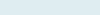# Sum Last N Values in a Column

When you have column and users want the count of values in the range and give the addition of last Nth values based on reference. The same way knows Average of last Nth values.

Query: We have two columns, Month and Total sales. Our task is to get total sales of the last five (5) months. For this, we will use Sum, Offset and Count function.

Formula to Implement:

=SUM(OFFSET(C3,COUNT(C3:C14)-5,0,5))

Functions applicable:

Sum: The Excel SUM function returns a number after adding multiple numeric arguments together.

Offset: The Excel OFFSET function returns one or multiple data points of a data set by providing a reference point of data set with a specified number of rows and columns to offset.

Count: The Excel COUNT function compute the count of numbers within the reference list of arguments ignoring the text and blank cells.

## Formula Explanation (step by step)

Excel starts calculating innermost parenthesis than work outward. You can also debug the formula using a shortcut F9 function key under the formulas tab.

Let’s break the formula into multiple small steps to understand its application.

First formula:

COUNT(C3:C14)

Syntax:  COUNT(value1, [value2], …)

Step 1: COUNT(7000;7600;5950;10752;9554;6028;7854;5570;8820;7040;9707;11207)

Result: 12

It returns “12” after counting numbers in the given range (C3 to C14).

Step 2: COUNT(C3:C14)-5

Result: 7

As we already get count 12 now after subtracting 5 we get 7.

Second Formula:

OFFSET(C3,COUNT(C3:C14)-5,0,5))

Syntax: OFFSET(reference, rows, cols, [height], [width])

Step 3: OFFSET(C3,7,0,5)

Result: C10:C14

It returns cells based on reference values of rows (7) and height (5) i.e. last 5 values (C10, C11, C12, C13 and C14) from the column.

Third Formula:

SUM(OFFSET(C3,COUNT(C3:C14)-5,0,5))

Syntax: =SUM(number1,[number2],…)

Step 4:Result: 42344

It returns after adding numbers (5570+8820+7040+9707+11207) in the given range (C10:C14).

Note: To know more about addition based on condition out How to use SUMIF with multiple conditions?